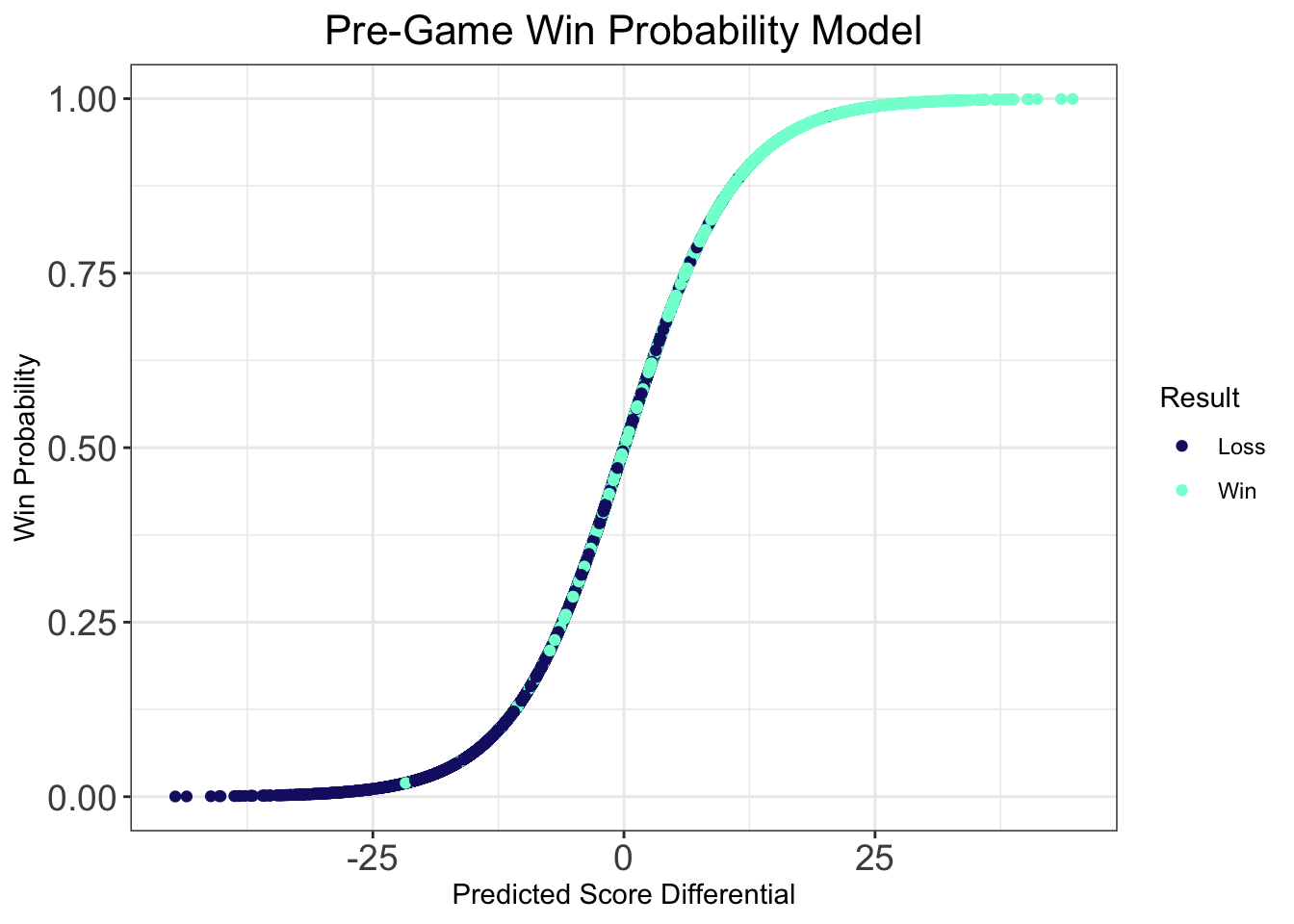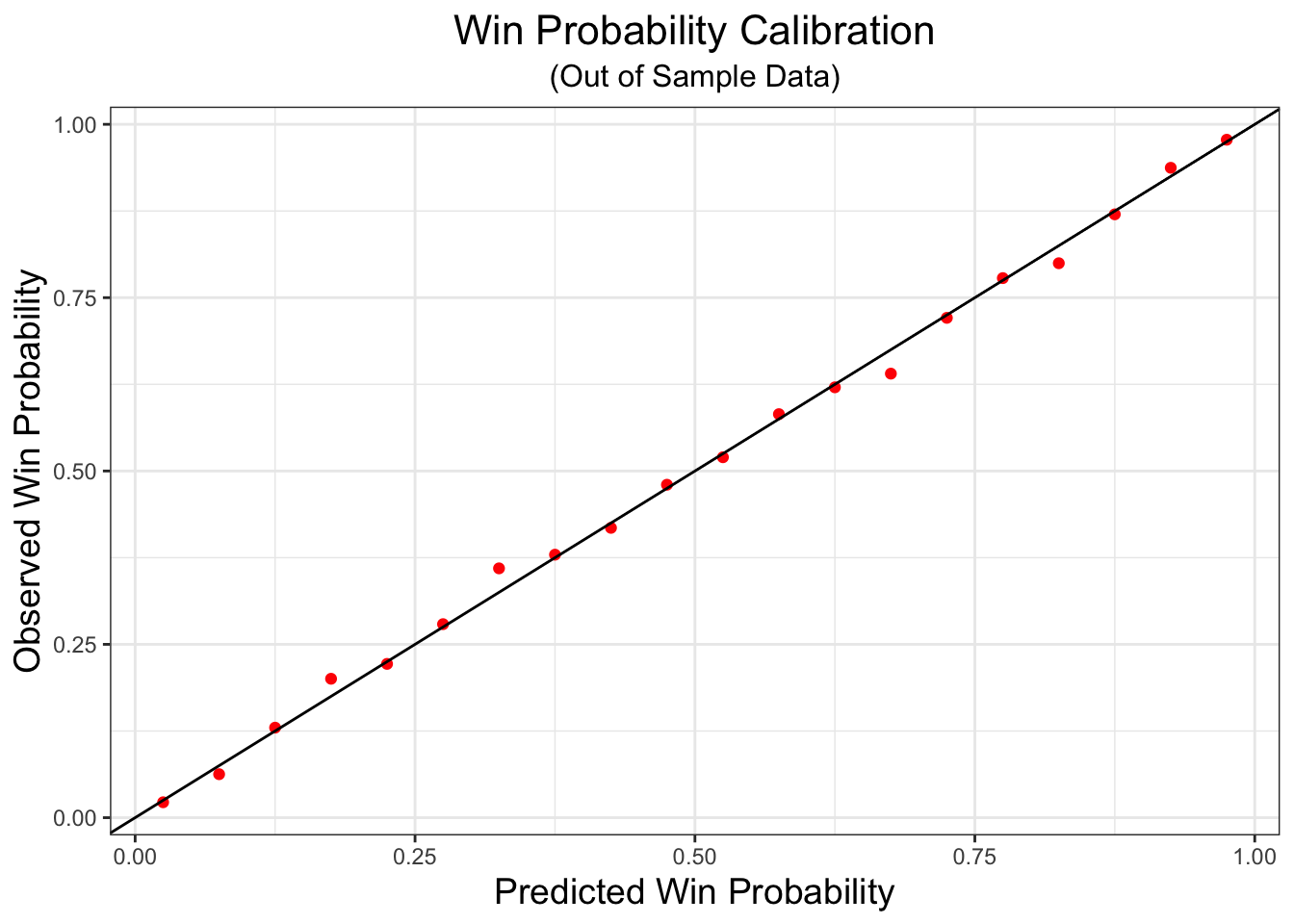# Introduction

For the past two years, I’ve been maintaining a men’s college basketball prediction model that I first built for the Yale Undergraduate Sports Analytics Group (YUSAG) during my sophomore year of college. The model has done objectively quite well over the past two seasons. It correctly predicted last year’s National Champion, Villanova, and accurately predicted North Carolina and Gonzaga as the most likely finalists in the 2017 title game. Brackets filled out using model derived predictions have finished above the 90th percentile on ESPN each of the past two seasons. Using my model, I even won the 2018 American Statsitical Association Statsketball Tournament. Having two seasons of experience with this project under my belt, there are a number of additional features I’ve been interested in adding/changing for the 2018-19 season.

# Background

Before we get into what’s different this year, we need to look at the way the YUSAG model has worked in the past. The core of the model is linear regression, specified by $Y = \beta_{team}X_{team} - \beta_{opp}X_{opp} + \beta_{loc}X_{loc} + \epsilon$

where $$X_{team, i}, X_{opp, i}$$, and $$X_{loc, i}$$ are indicator vectors for the $$i^{th}$$ game’s team, opponent, and location (Home, Away, Neutral) from the perspective of team, and $$Y_i$$ is game’s the score-differential. The key assumptions for this model are that game outcomes are independent of one another, and that our error $$\epsilon \sim N(0, \sigma^2)$$.

$$\beta_{team}$$, nicknamed “YUSAG Coefficients”, were scaled to represent the points better or worse a team was the average college team basketball team on a neutral court. Lastly, $$\beta_{loc}$$ is a parameter indicating home-court advantage, estimated to be about 3.2 points.

Note that the coefficients $$\beta_{opp}$$ have the same values and interpretation as $$\beta_{team}$$. I’ll note that when this model is actually fit, $$\beta_{opp} = -\beta_{team}$$ but in the interest of easy interpretation in these methodology notes, I have flipped the signs of the $$\beta_{opp}$$ coefficients and added a minus sign to the model formulation above.

Let’s walk through an example to see how this all works. Say Yale is hosting Harvard, and we’d like to predict score differential. $$\widehat\beta_{team = Yale} = -2.1$$ and $$\widehat\beta_{opp = Harvard} = 1.9$$. This means that on a neutral court, Yale is 2.1 points worse than the average college basketball team, and Harvard is 1.9 point better. Our predicted outcome for this game would be as follows: $\widehat Y_i = \widehat \beta_{team = Yale} - \widehat \beta_{opp = Harvard} + \widehat \beta_{loc = Home} = -2.1 - 1.9 + 3.2 = -0.8$ Hence, we’d expect Harvard to win this game by roughly 0.8 points. Of course, we could’ve predicted the game from the perspective of Harvard as well, and we’d get exactly the same answer. Since $$\beta_{opp = Yale} = \beta_{team = Yale}$$ and $$\beta_{team = Harvard} = \beta_{opp = Harvard}$$, we have $\widehat Y_i = \widehat \beta_{team = Harvard} - \widehat \beta_{opp = Yale} + \widehat \beta_{loc = Away} = 1.9 - (-2.1) - 3.2 = 0.8$

We recover the Harvard by 0.8 predicted scoreline, and hence, it doesn’t matter which school we use as “team” or “opponent” because the results are identical. Once we have a predicted score differential, we can convert this to a win probability using logistic regression. I won’t get into the specifics how logistic regressions works in this post, but for the purposes of this example, just think about it as a translation between predicted point spread and predicted win probability.One thing that we check to see is that the win probability model is well calibrated. That is, if we predict a team has a 75% chance of winning, then we should see them win about 75% of the time on out of sample data. To see that the model is well tuned, I’ve fit the logistic regression on data from the 2017-18 season, and then made predictions of win probability from prior predicted score differentials for the 2016-17 season. We don’t see any drastic deviations from the line denoting perfect correspondence between predcited and observed win probability. This is good–it means teams are winning about as often as we predict they are winning!# Offense and Defense Specific Models

Now that we have the basics of model, we can dive into what’s new for this season. In the past, I’ve only been interesting in predicting game score differential, $$Y_i$$. In fact, we can rewrite score differentials as $$Y_i = T_i - O_i$$, where $$T_i$$ denotes team_score and $$O_i$$ denotes opp_score for game $$i$$, respectively. Not only is the distribution of score_differential normally distributed, but so too is the distribution of team_score. I’ll note that the gap around 0 in the distribution of score_differential is due to the fact that games can’t end in ties. I’ll also comment that the distribution of opp_score is identical to that of team_score as each game is entered twice in the database, so there is a direct one-to-one correspondence between each value of team_score and opp_score.